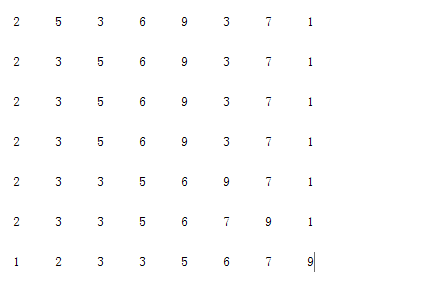matlab 程式碼如下

%%Insertion-sort:插入排序
A=[2 5 3 6 9 3 7 1]; %%輸入待排序序列
for j=2:length(A)
key=A(j);
i=j-1;

while i>0&& A(i)>key %插入排序內迴圈
A(i+1)=A(i);
i=i-1;
end
A(i+1)=key;

disp(A); %分別輸出每次排序後的結果
end%%Insertion-sort:插入排序   時間複雜度分析      次數
A=[2 5 3 6 9 3 7 1];
for j=2:length(A)                           % n：從2到length（A)是n-1次，當一個for或while迴圈按通常的方式退出時，執行測試的次數比迴圈體的次數多1次
key=A(j);                               %n-1
i=j-1;                                  %n-1
while i>0&& A(i)>key                    %t1
A(i+1)=A(i);                        %t2
i=i-1;                              %t3
end
A(i+1)=key;                             %n-1
disp(A);
end
t1=j=2ntj
t2=j=2n(tj1)
t3=j=2n(tj1)

T(n)=c1n+c2(n1)+c4(n1)+c5j=2ntj+c6j=2n(tj1)+c7j=2n(tj1)+c8(n1)

1)執行時間分析： 插入排序時間開銷與輸入序列的規模（即待排序陣列的長度）及陣列的已排序程度有關。輸入序列規模越大，時間開銷越大；相同規模的兩個序列，已排序程度越小，時間開銷越大。演算法時間開銷的最佳情況出現在輸入序列為已排序序列時，最壞情況出現在輸入序列為逆序排列序列時。

2)時間複雜度：Θ(n^2)（注：時間複雜度一般考察演算法的最壞情況時的時間代價）。

3)插入排序是原地排序（sort in place），即在待排序的陣列內部進行排序，不需要額外申請新的空間作臨時容器。

4)插入排序是穩定排序（stable sort），所謂穩定排序是指待排序序列中有兩個（或者兩個以上）相等的元素：Ai=Aj，排序前i